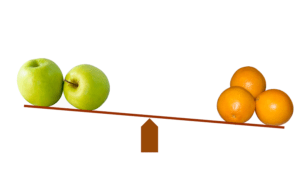Want to share your content on R-bloggers? click here if you have a blog, or here if you don't.In this post I explore two different methods for computing the relative importance of predictors in regression: Johnson’s Relative Weights and Partial Least Squares (PLS) regression. Both techniques solve a problem with Multiple Linear Regression, which can perform poorly when there are correlations between predictor variables.

When there is a very high correlation between two predictor variables, Multiple Linear Regression can lead to one of the variables being found to be a strong predictor, while the other is found to have a relatively small effect. Relative Weights computes importance scores that factor in the correlation between the predictors. The goal of PLS is slightly different. It is designed to work in situations where it is impossible to get stable results from multiple regression. Instability may be because of extremely high correlations between variables (aka multicollinearity), or where there are more predictor variables than observations.

Relative Weights is my “go to” method in situations where there are non-trivial correlations between predictor variables (click here to read more about some of its strengths). However, in some fields, such as chemometrics and sensory research, PLS is the “go to” method because they have more predictor variables than observations. In this post I seek to understand how similar the techniques are when applied to a data set with moderate correlations.

# The case study

The data set I am using for the case study contains 34 predictor variables and 1,893 observations. I use it to look at the relationship between 327 consumer’s perceptions of six different cola brands. Click here to read more about this case study. The correlations between the variables are shown below.

# Comparing the methods

The chart below shows the relative importance computed using Johnson’s Relative WeightsPLS,  and Multiple Linear Regression. (Click here to see how to estimate PLS in R and here to see how to do this in Displayr.) In contrast to this earlier case study using this data, I am showing negative effects for Multiple Linear Regression. I use the same signs for Relative Weights because Relative Weights does not distinguish between whether the effect is positive or negative.

The chart shows us that there is a strong correlation between each of the methods. However, that should not be a great surprise, as they all use the same data and have the same functional form (i.e., linear with additive effects).

The R2 statistic computed predicting the PLS coefficients by the Multiple Regression coefficients is 0.98. I fitted this model without an intercept. This demonstrates that the Multiple Linear Regression and PLS results are very similar.

The R2 between the PLS and the Relative Weights is 0.85, which tells us that PLS is much more similar to Multiple Linear Regression than to Relative Weights. The Rbetween Multiple Linear Regression and the Relative Weights is 0.81, which means that PLS is somewhere in between the two other techniques, but is much more similar to Multiple Linear Regression.

If we look at the chart we can see some striking differences between Relative Weights and the other two methods:

• Health-conscious is much less important for Relative Weights than in the other analyses.
• All the negative importance scores are much closer to 0 for Relative Weights than for the other analyses.
• The small positive importance scores are greater for Relative Weights than for the other analyses.

# Rescaling and removing the negative scores

The above analysis shows that we get large and meaningful differences between Relative Weights and PLS. Why? One explanation relates to standardization. Relative weights automatically standardizes the predictor variables, presenting the importance scores in terms of their ability to explain R2PLS does not standardize the predictors. A second explanation relates to negative coefficients. Relative Weights ignores the sign of coefficients, with the signs for the Relative Weights in the above analyses derived from a separate Multiple Regression. To appreciate the effect of these two things, I:

• Standardized all the variables (i.e., divide each variable’s raw data by the variable’s standard deviation).
• Re-estimated all of the models, but only used variables with positive coefficients.

The coefficients are plotted below. They are a lot more similar than in the previous analysis. The PLS and Multiple Linear Regression,  are particularly close, with an R2 of 0.998. The R2  for the PLS versus the Relative Weights is 0.95. For the Relative Weights versus Multiple Linear Regression it is 0.94. Given each analysis is for the same data set and the same core assumptions, 0.94 is still quite a difference.

Looking at the chart, we can see that the conclusions from the models are substantively different. In particular:

• Relative Weights tends to conclude that the relatively unimportant variables are more important than is the case with either PLS and Multiple Linear Regression. This can be seen from the bars at the very bottom of the chart.
• The conclusions of the models regarding Health-conscious remain striking. The Multiple Regression and PLS both conclude that is the 10th most important variables. By contrast, Relative Weights has it as the least important variable. This earlier case study discusses the reasons for the difference.

# Conclusion

Although PLS and Johnson’s Relative Weights are both techniques for dealing with correlations between predictors, they give fundamentally different results. In this data set, where the correlations are moderate, PLS is little different from Multiple Linear Regression. By contrast, Relative Weights gives substantially different conclusions.

TRY IT OUT

All the analysis in this post was conducted using R in Displayr. Click here to review the underlying data and code, or to run your own analyses.